## Fluids

•ywang2524
Subscriber
Dear all,n I am trying to simulate the thermal performance of a short part of the shell side of a helixal-bundled-tubes heat exchanger, the objective is to obtain the friction factor and Nu value under different Re values. I am using k-omega model, velocity inlet and pressure outlet, helium as working fluid, and constant wall flux boundary condition.n When I used steady model, the case diverged (absolute residuals oscillate and then increase before reaching 10e-3), then I choose transient model without any other setting changed, the case could converge well (absolute residuals reach 10e-4 and continuely decrease and then become stable in every time step) and I can see more details in the velocity profile when postprocessing.n I suppose the transient model will reach steady state after enough time steps. In my case, what is the reason of the different results of using steady model and transient model? Thanks a lot!n Best regards.
•Rob
Ansys Employee
I'd expect the transient result to tend towards the steady in this case assuming both converge. If steady is oscillating and then diverging I suspect you have picked up a transient in the flow field. What does the flow field look like and how are the point monitors behaving in the steady case? n
•ywang2524
Subscriber
Thanks for your reply! Could you explain what does pick up a transient in the flow field mean? nThe flow field in the steady case,n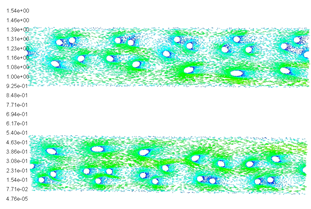The flow field in the transient case,n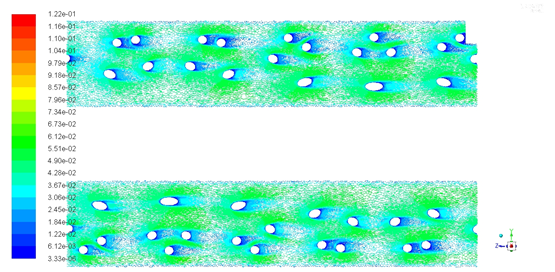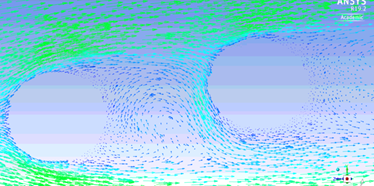There are eddies behind each tube in the transient case while the result of steady case does not show the eddies (even under a larger Re value).nI did not set point monitor, is it helpful? Thanks a lot.n
•Rob
Ansys Employee
In your model the cylinders are creating a wake. That then interacts with the downstream cylinder and it's wake. Read up on von Karman vortices and Coanda Effect. That will be inherently transient. In steady the solver is trying to work out which of the many combinations is the equilibrium state and failing: I'd normally expect poor convergence rather than a complete divergence in this scenario. nMonitors are covered in the tutorials and we use them to check for convergence in some cases: you plot a variable on a point/surface/volume with iteration (steady state) or time step (transient) to get more information on the flow field. n
•ywang2524
Subscriber
Thanks very much! So how the cylinder interacts with the downstream cylinder? I am using the pressure outlet and set the backflow temperature, is this the setting what matters the interaction?n
•Rob
Ansys Employee
Nothing to do with the backflow: it's the wakes of the obstructions that you've highlighted in the last image. I thought they were cylinders: it's the helix cross section.... n
•ywang2524
Subscriber
OK I get it, yes the cylinders you mean are the helical inner tubes, I thought you were talking the whole geometry. Thank you!n
•YasserSelima
Subscriber
Von Karman or vortex shedding occurs behind bluff body in a flow .. something like what you see here in the photo of an island in ocean n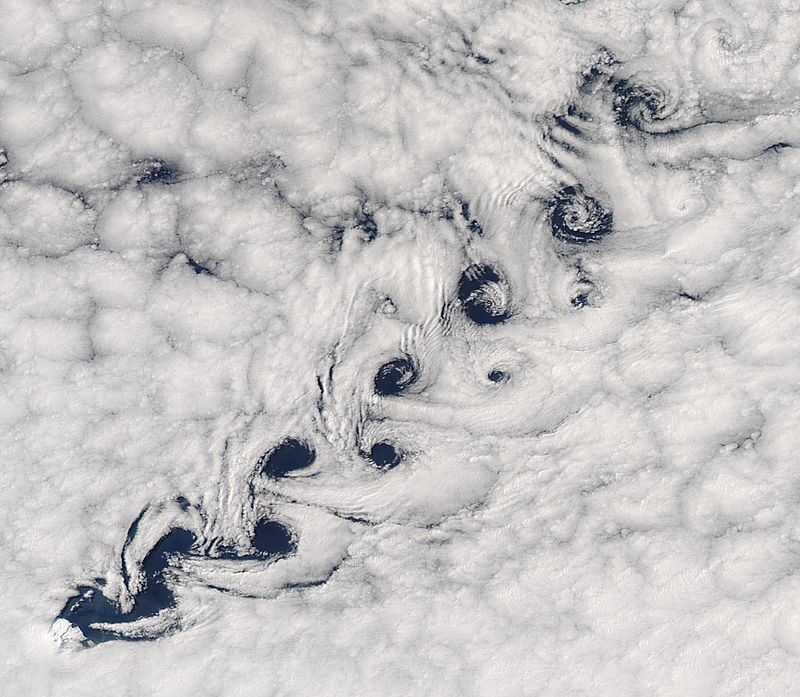nThese vorticies alternate up and down. When they happen on the upstream cylinders, this affect the velocity profile on the downstream ... That is why for heat transfer in heat exchanger, the Nu number is different in the first two rows than the rest. Helical tubes in your case would break this vortex, but not completely ... So, I would definitely go with suggestion and trust the unsteady simulation.nAs you are interested in Nu number, you can create an expression to calculate Nu on each tube. Then you can plot a relation between Nu and the flow time. This what we call monitors ... and wait until Nu values are almost constant, or periodic .. and now it is steady state. nThen you can do the study by yourself comparing Nu in the steady state solution to that obtained in and unsteady solution and see the difference.n
•ywang2524
Subscriber
Thanks for your suggestion, I will try to get the plot between Nu and the flow time. For the calculaiton of the Nu, I did not use the area weightd average surface heat transfer coefficient calculated by fluent postprocessing since I found it would change sharply even with a small change of reference temperature and it's hard to identify the reference temperature. I used the difference of the mass weighted average temperature of the interior flow part and average temperature of the wall to calculate the heat transfer coefficient, then the Nu value, does it make sense?n
•YasserSelima
Subscriber
It makes sense as you are monitoring and interested to see when you reach the steady state. n
•Rob
Ansys Employee
as the HTC is a derived term you just need to be consistent between the model and whatever the design rules or literature state. I tend to use the heat flux combined with mean bulk temperature difference (shell side to tube side) but rarely need to provide a HTC as the fluxes or wall temperature are what we are interested in. n
•ywang2524
Subscriber
nDear all, for the transient problem, I met the divergence problem again. The case converged well in the first hundreds of time steps, then the continuity residual or the K value (I am using K-omega model) residual can not reach the convergence standard and finally diverged. I have tried different inlet velocity and tempreature, under each condition I set the time step a different value to satisfy the courant number a bit smaller than 0.5. Is it possible that the velocity at some area in the flow field will be larger than the inlet velocity due to the vortices, which lead to a large courant value and divergence? I used the implicit method, shouldn't it be stable no matter how large the courant value is? Thanks so much!nCase converged in 43 iterations in the 201 time steps, and then can not converge in the following time steps:n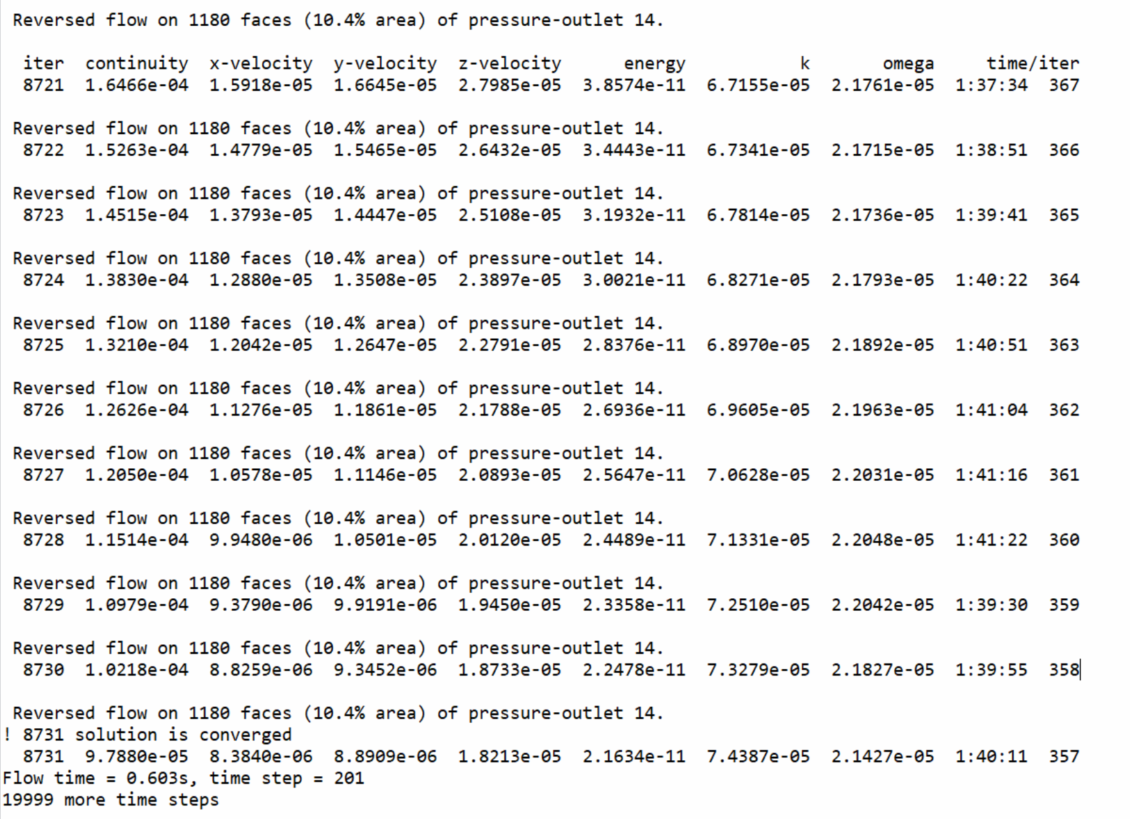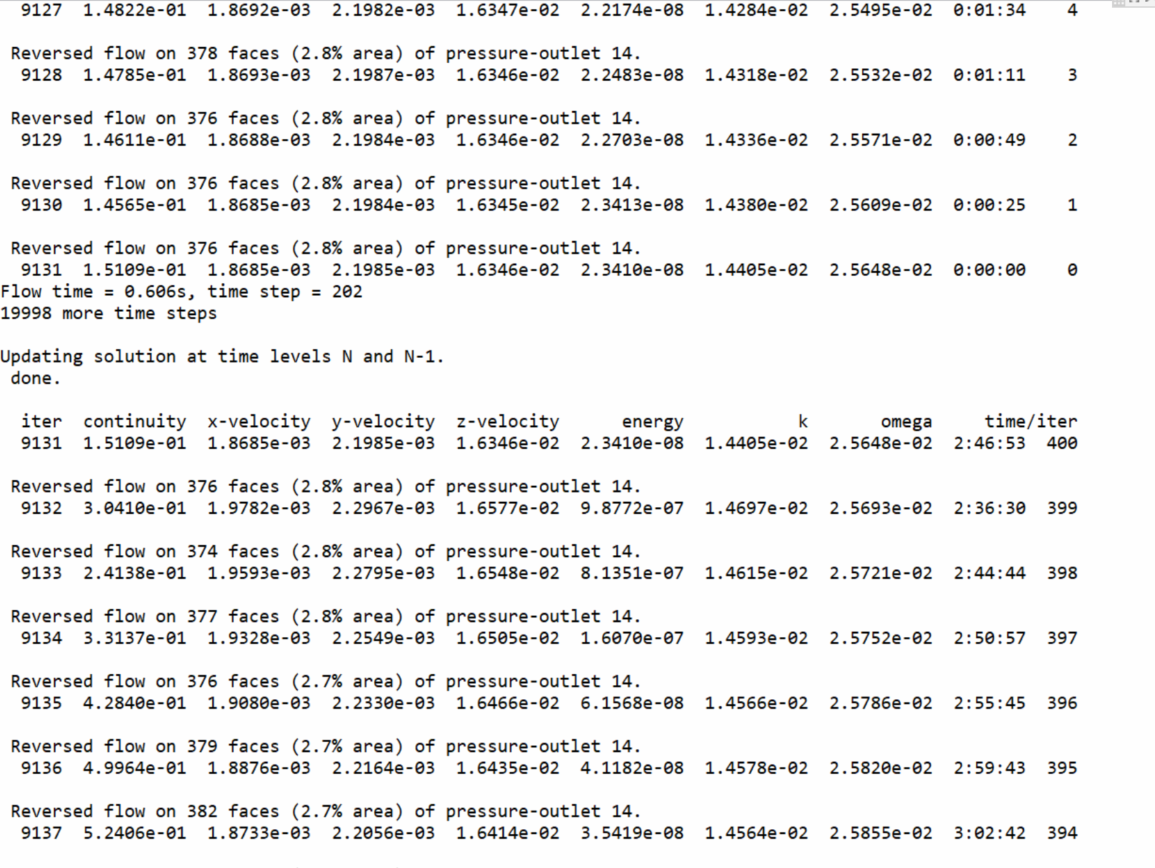•Rob
Ansys Employee
Have a look at the flow field and see what's changing. If you've got inflation mesh have you found a condition where the flow is crossing a cell therefore requiring the time step to be even smaller?n
•ywang2524
Subscriber
Thanks for your reply! Yes I realized that the mesh around the inner tubes are much smaller than the others, so I should use the smallest cell size to calculate the time step?n
•Rob
Ansys Employee
That may be necessary. The cell size / flow velocity / 10 is a rough guide, if the model doesn't converge well decrease the time step. But always check what's going on to see if there's a flow feature you need to account for. n
•ywang2524
Subscriber
Ok, thanks very much!n
•ywang2524
Subscriber
Hello again! The convergence problem still exists, I have decreased the time step from 0.005 s to 2e-7 s, every time I decreased the time step, the case moved forward some steps then diverged again. I also tried decreasing the under relaxation factor. I monitored the velocity and temperature values at several points, and found that the temperature stays the same while the velocity values of all points change smoothly in the converged steps and then increase sharply, and then the case diverges. Any idea what is the cause of the sudden change of velocity? Thanks so much!n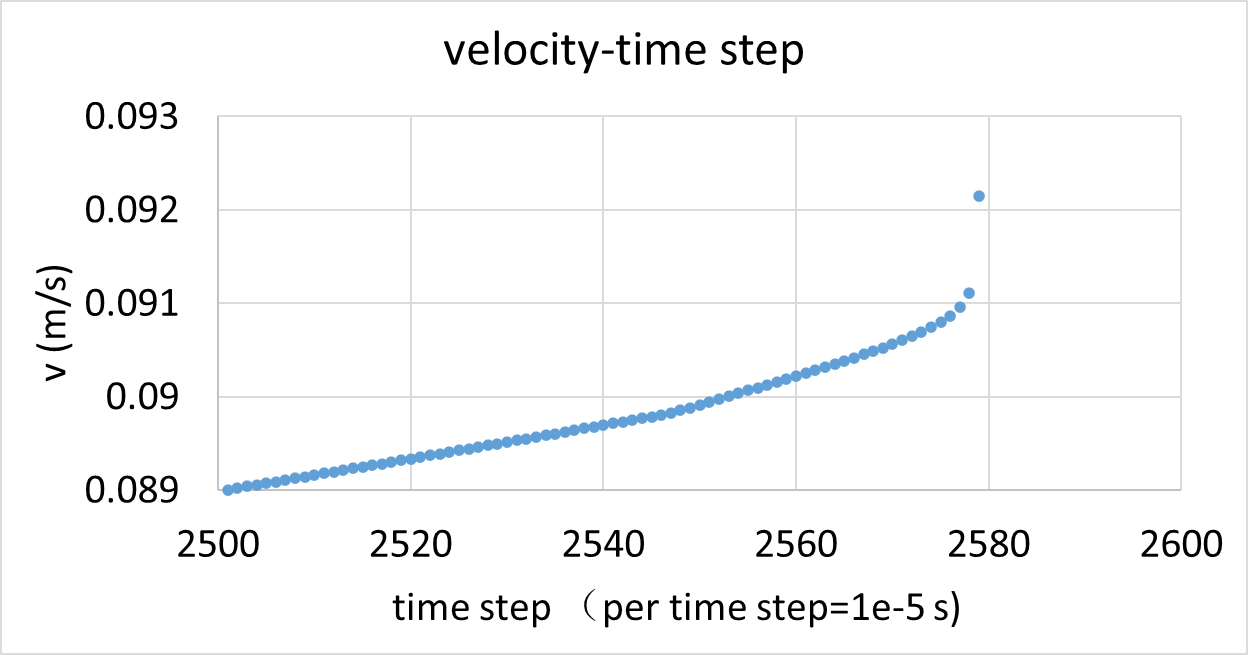n
•Rob
Ansys Employee
Going back to an earlier comment. What is happening in the flow field just before the jump in velocity. You need to review vectors and contours, just plotting a monitor isn't always very diagnostic. n
•ywang2524
Subscriber
Sorry for the late reply, the high performance cluster in my university is really crowded these days and it takes several days to run my case. I found that the velocity distribution becomes more and more ununiform. Following are the velocity distribution at the surface x=0, you can see the maximum velocity becomes larger and larger with time while the minimum velocity becomes smaller and smaller.n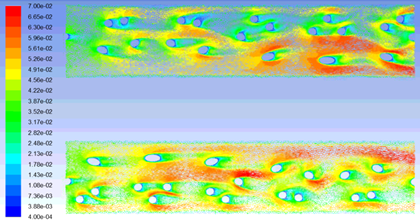t=1.25 s                                                                     n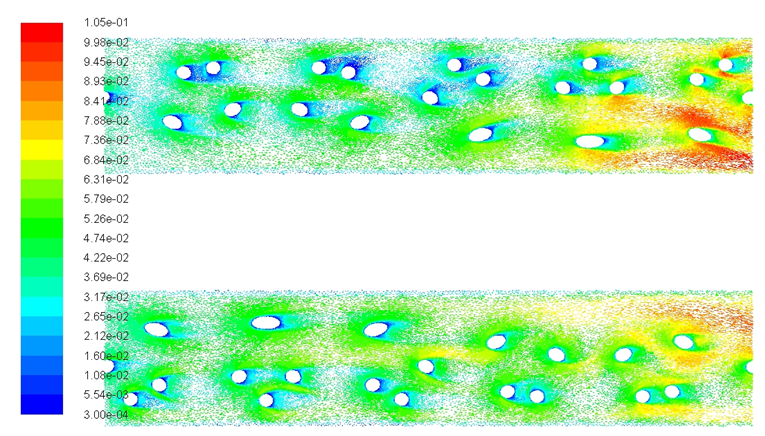t=1.292627 snn  The velocity distribution at the surface y=0 looks similar as that at the surface x=0 at t=1.25 s, while the distributions look quite different at t=1.292627 s, which is the last converged time step. n  n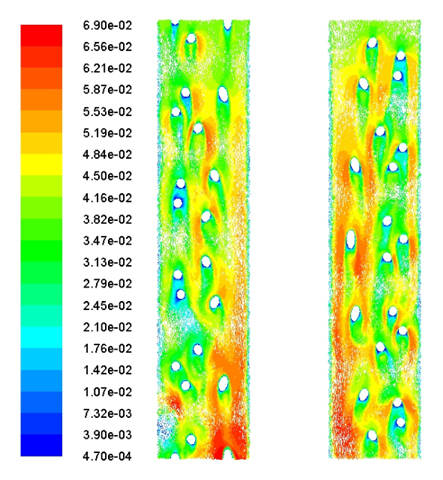t=1.25 s                                                                    n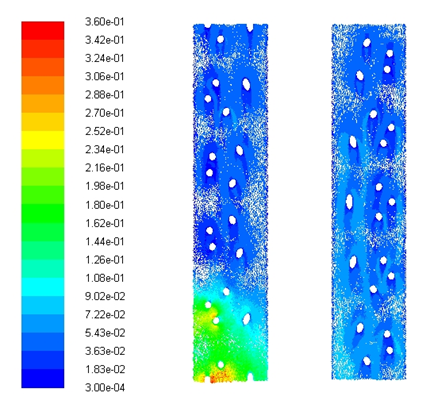t=1.292627 sn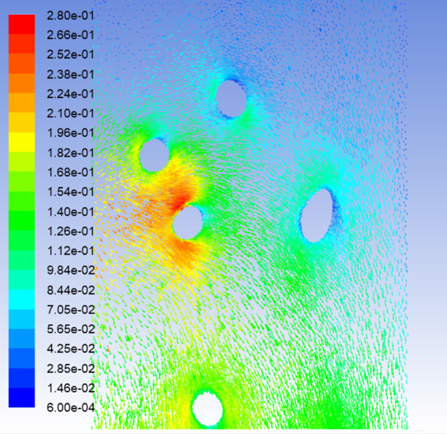some details of the high velocity area at t=1.292627 snn   We have considered that maybe the cause is the low viscosity of helium under low temperature (30 K), however I have tried to set a large viscosity with all other parameters unchanged, and it did not solve the problem. We would be appreciated if you have any advice. Thanks so much!nn
•Rob
Ansys Employee
That may be diverging, ie the solver is failing for some reason. Can you check the mesh quality in that region (cell quality and mesh resolution). Do you start to get back flow warnings at some point in the model? Are you converging every time step? There's a way of monitoring number of iterations per time step, look in reports. n
•ywang2524
Subscriber
The mesh in that region is relatively coarse comparing with that around the inner tubes. The minimum orthogonal quality is 0.17, and there are 18 million cells in the whole 50 mm long domain. I do get back flow warnings all the time, but the back flow area are becoming smaller and smaller with time. There are reversed flow on 10.4% area at 0.603 s, 4.7% area at 1.2545 s, and 1.2% area at 1.2926204 s. The residuals reach the convergence stardard I set every time step before diverging, and I also tried decreasing the convergence standard. Does the reversed flow information look strange? Thanks.n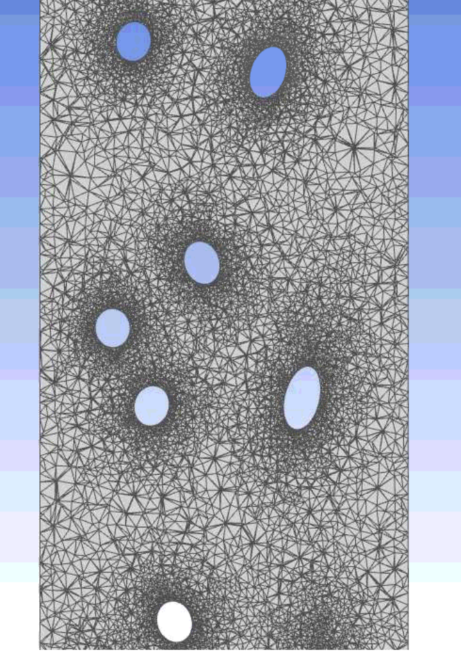n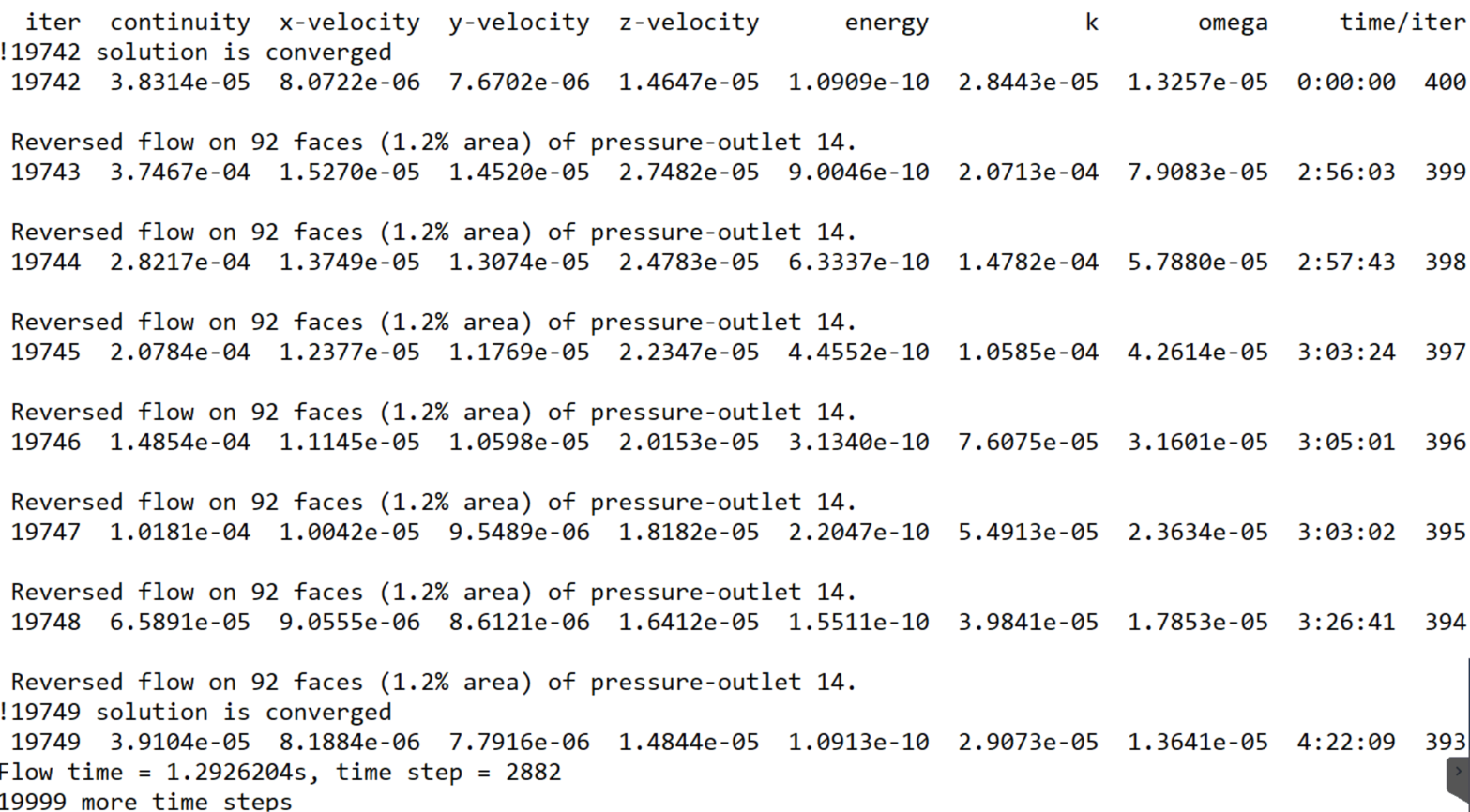n
•Rob
Ansys Employee
That looks fine, and the residuals also look good. What density model are you using? n
•ywang2524
Subscriber
I am using a constant density since there is only 1 K temperature change.n
•Rob
Ansys Employee
What solver settings are you using in steady? n
•ywang2524
Subscriber
•YasserSelima
Subscriber
At this low temperature, it is expected that the velocities go high at few cells, specially around the tubes. decrease the time step further and run few time steps and see if the overall solution changes or not.n
•ywang2524
Subscriber
Ok I will try, thanks!n
•ywang2524
Subscriber
Hi, I decreased the time step further to 5e-8 s and run several time steps, the overall solution does not get better. The velocity distribution on surface x=0 seems more normal, the velocity at most area is around 0.03 m/s, which is the inlet velocity. However, the velocity distribution on surface y=0 is abnormal, the velocity at most area is much smaller than the inlet velocity, and then increases sharply at the area near the outlet. Surface x=0 and surface y=0 are two vertical slices of the whole domain, why do they behave so different? Thanks so much!nvelocity distribution on surface y=0n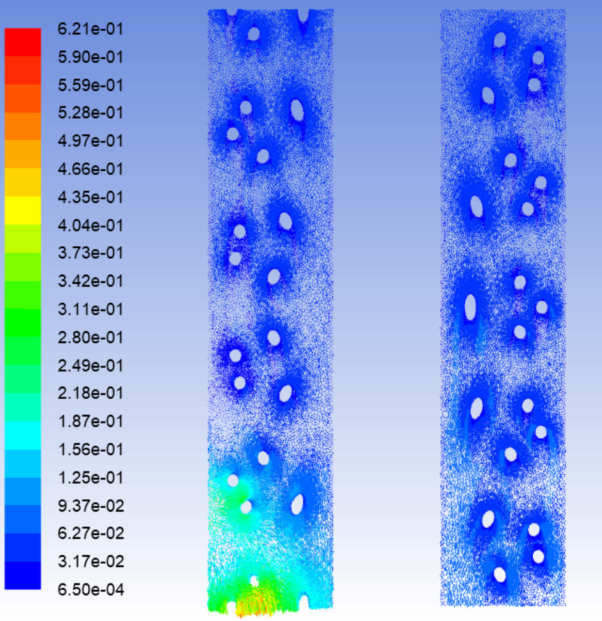nvelocity distribution at surface x=0n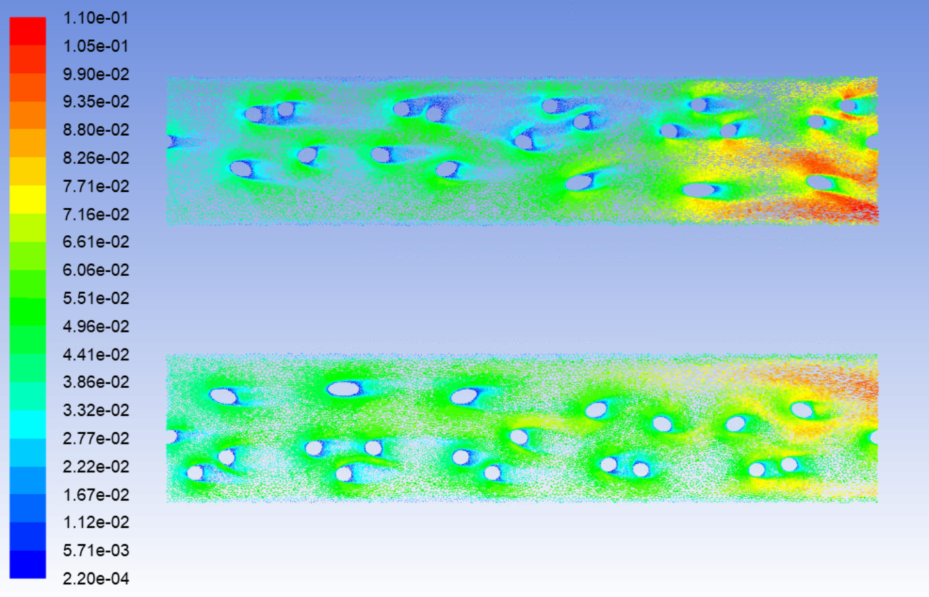n
•Rob
Ansys Employee
Can you turn on Higher Order Term Relaxation, HOTR. You'll need to go back a few saves to where the high velocity hasn't stated. It may be a case that the solution isn't stable and the higher order scheme needs some help. n
•ywang2524
Subscriber
Hi, I turned on HOTR and it does help, now I can arrive at 1.3 s using a time step 10e-4 s and still wait for more results, so could you please explain more about how HOTR helps? Thanks a lot!n
•Rob
Ansys Employee
It's a blending function, so where the solution is very stiff it'll relax to a nearer first order solution. In the old days we started all models with first order discretisation and if the convergence was good would try second (or similar) order to improve the accuracy. With the newer solvers we just launch with higher order and use HOTR to help with convergence. n
•ywang2524
Subscriber
Does this kind of solution provide more detail? The size of data file increases from 5 GB to 10 GB...... n
•Rob
Ansys Employee
No, it just tends to converge better. I rarely check the data file size so hadn't noticed. n